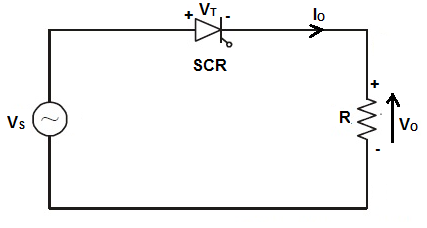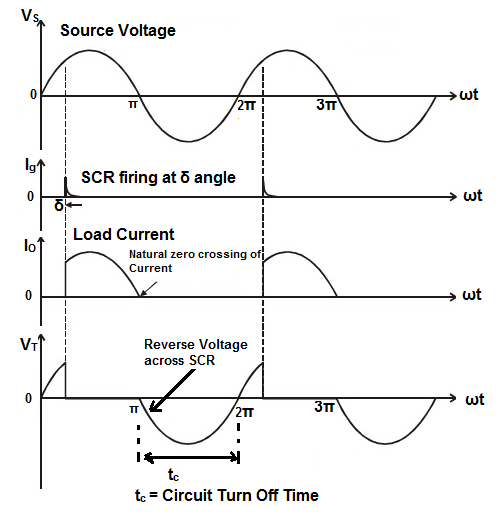# Commutation of SCR and its Types

Commutation in Power Electronics refers to the process of commutation of SCR. Whenever we talk of commutation in power electronics, we simply mean the process to turn off an SCR. This article details about the commutation of SCR, Natural Commutation, Forced Commutation and various types of Forced Commutation techniques.

## What is Commutation of SCR?

Commutation of SCR is defined as the process of turning off an SCR / thyristor.  It is the process by which an SCR or thyristor is brought to OFF state from ON state. We know that, an SCR is turned on by applying a gate signal to a forward biased SCR. Please read “Required Conditions to Turn-on an SCR” for better understanding. But for the purpose of power control or power conditioning, it is required to turn off SCR as and when required. Turn off of a thyristor means bringing it to forward blocking mode from forward conduction mode. We also know that, once an SCR goes in forward conduction mode, gate loses its control. This means, some external techniques / circuit must be employed to turn off SCR. This external circuit is known as commutation circuit.

To turn off an SCR / thyristor, it is required that its anode current should fall below the holding current and a reverse voltage should be applied across the SCR for the sufficient time so that it regains to forward blocking mode from forward conduction mode. Thus, to turn off an SCR some methods must be applied so that the above mentioned conditions can be met to turn off SCR as per requirement. This method of turning off an SCR / thyristor is called commutation process.

## Types of SCR Commutation Techniques:

There are mainly two types of SCR commutation techniques: Natural Commutation and Forced Commutation.

### Natural Commutation of SCR:

Natural Commutation of SCR is the process of turning off an SCR without using additional commutation circuitry.  This commutation technique only occurs in AC circuit.  For better understanding, let us consider an SCR circuit energized from AC source.When SCR is conducting, the current will pass through zero after every positive half cycle. After that, the AC source then applies a reverse voltage across the terminals of SCR till the beginning of second cycle. If the time of application of reverse voltage applied by the AC source is more than the SCR turn-off time, then SCR will get turned off.From the above waveform, following points can be noted:

1. SCR is fired or gated at an angle of δ on the Source Voltage.
2. The waveform of output current or SCR anode current (Io = Vo / R) is sinusoidal as the load is resistive load.
3. When the AC Source Voltage reaches zero at ωt = π, the load current or SCR anode current becomes zero.
4. After ωt = π, the source voltage becomes negative and hence, the voltage across SCR also becomes negative. Thus SCR is reversed biases from ωt = π to ωt = 2π i.e. for a time period of tc = (π / ω).  This time tc is called Circuit Turn Off time
5. If  tc is more than the SCR turn off time, then SCR will get turned off.

Thus we see that, the SCR is getting turned off due to the natural tendency of AC. This is the reason, this type of commutation technique is called Natural Commutation. It should also be noted that, no additional circuitry is required in this commutation technique. This commutation technique is basically the Class-F commutation (you might encounter in many literature) technique which is also known as Line Commutation of SCR.

### Forced Commutation of SCR:

Unlike natural commutation, an external circuitry is required to forcibly bring the SCR andoe current below holding current and keeping SCR reversed biased for a period more than the SCR / thyristor turn off time. This technique is applied for DC circuit. The commutation circuitry for forced commutation comprises of Inductor and capacitor. Forced commutation is applied to Choppers and Inverters.

There are several forced commutation techniques. They are as follows:

• Class-A Commutation (also known as Load Commutation)
• Class-B Commutation (also known as Resonant Pulse Commutation)
• Class-C Commutation (often called Complimentary Commutation)
• Class-D Commutation or Impluse Commutation
• Class-E Commutation or External Pulse Commutation

This site uses Akismet to reduce spam. Learn how your comment data is processed.